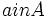# SCDIN-subgroup

## Contents

BEWARE! This term is nonstandard and is being used locally within the wiki. [SHOW MORE]
This article defines a subgroup property: a property that can be evaluated to true/false given a group and a subgroup thereof, invariant under subgroup equivalence. View a complete list of subgroup properties[SHOW MORE]

## Definition

### Symbol-free definition

A subgroup of a group is termed a SCDIN-subgroup, or subset-conjugacy-determined in normalizer, if it is a subset-conjugacy-determined subgroup inside its normalizer, relative to the whole group.

### Definition

A subgroup$H$ of a group$G$ is termed a SCDIN-subgroup, or subset-conjugacy-determined in normalizer, if, whenever$A,B$ are subsets of$H$ such that there exists$g \in G$ with$gAg^{-1} =B$, there exists$k \in N_G(H)$ such that$kak^{-1} = gag^{-1}$ for all$a in A$.

## Relation with other properties

### Stronger properties

Property Meaning Proof of implication Proof of strictness (reverse implication failure) Intermediate notions
direct factor
central factor
retract
subset-conjugacy-closed subgroup subset-conjugacy-determined in itself
normal subgroup
Sylow TI-subgroup Sylow and TI implies SCDIN
Abelian pronormal subgroup Abelian and pronormal implies SCDIN
Abelian Sylow subgroup Abelian and Sylow implies SCDIN

### Weaker properties

Property Meaning Proof of implication Proof of strictness (reverse implication failure) Intermediate notions
NSCDIN-subgroup
CDIN-subgroup
WSCDIN-subgroup
WNSCDIN-subgroup
MWNSCDIN-subgroup